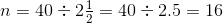# SSAT Upper Level Math : How to find the ratio of a fraction

## Example Questions

### Example Question #1 : How To Find The Ratio Of A Fraction

A pie is made up ofcrust,apples, andsugar, and the rest is jelly. What is the ratio of crust to jelly?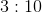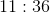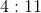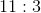Explanation:

A pie is made up ofcrust,apples,sugar, and the rest is jelly. What is the ratio of crust to jelly?

To compute this ratio, you must first ascertain how much of the pie is jelly. This is: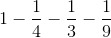Begin by using the common denominator: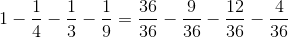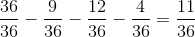So, the ratio of crust to jelly is: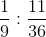This can be written as the fraction: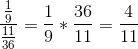, or### Example Question #1 : How To Find The Ratio Of A Fraction

In a solution,of the fluid is water,is wine, and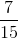is lemon juice. What is the ratio of lemon juice to water?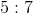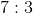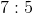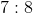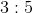Explanation:

This problem is really an easy fraction division. You should first divide the lemon juice amount by the water amount: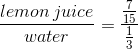Remember, to divide fractions, you multiply by the reciprocal: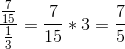This is the same as saying:### Example Question #5 : How To Find The Ratio Of A Fraction

If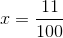and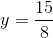, what is the ratio ofto?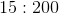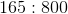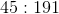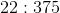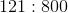Explanation:

To find a ratio like this, you simply need to make the fraction that represents the division of the two values by each other. Therefore, we have: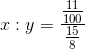Recall that division of fractions requires you to multiply by the reciprocal: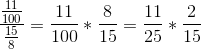which is the same as: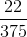This is the same as the ratio:### Example Question #31 : Proportion / Ratio / Rate

A smoothie is made up of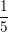pineapple juice and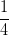mango juice; the rest is orange juice. What is the ratio of orange juice to pineapple juice?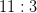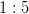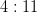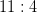Explanation:

First, find out how much of the smoothie is orange juice.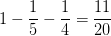Since we want the ratio of orange juice to pineapple juice, we want to set up the fractions like this: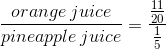Now, divide these fractions.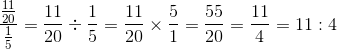### Example Question #32 : Proportion / Ratio / Rate

A bakery sells three different types of cookies.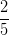of the cookies sold are chocolate chip,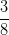sold are oatmeal raisin, and the rest sold are sugar cookies. What is the ratio of sugar cookies to oatmeal raisin cookies that the bakery sells?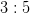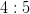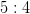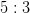Explanation:

First, find the fraction of sugar cookies that are sold.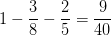Since we want the ratio of sugar cookies to oatmeal raisin cookies: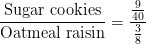Now, multiply and simplify the resulting fraction.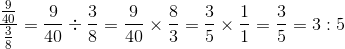### Example Question #1 : How To Find The Ratio Of A Fraction

What number can be filled in the blank to make the two ratios equivalent?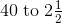________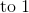Explanation:

Set up a proportion statement: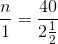Rewrite: